Question

Carry out analysis of a beam hanging under its own weight in a 1d space using the finite element 10-step procedure. Carry out the analysis using a single 1d quadratic element. The figure below contains the properties for the beam.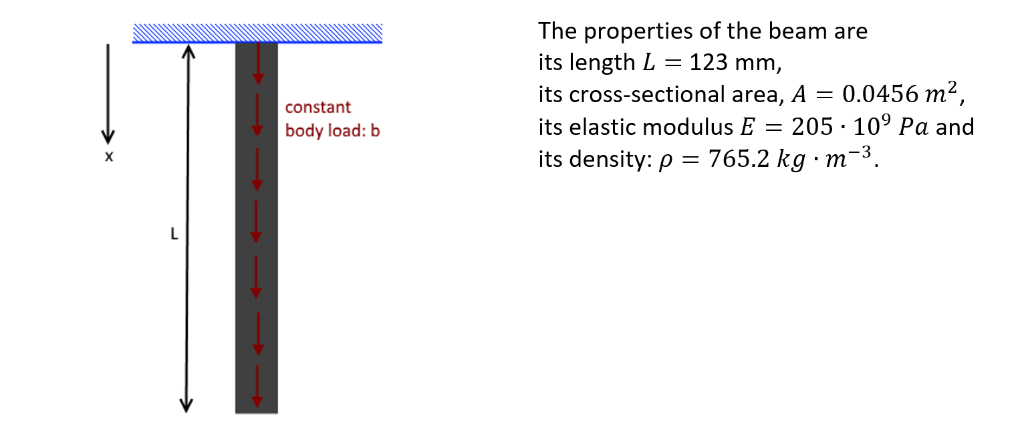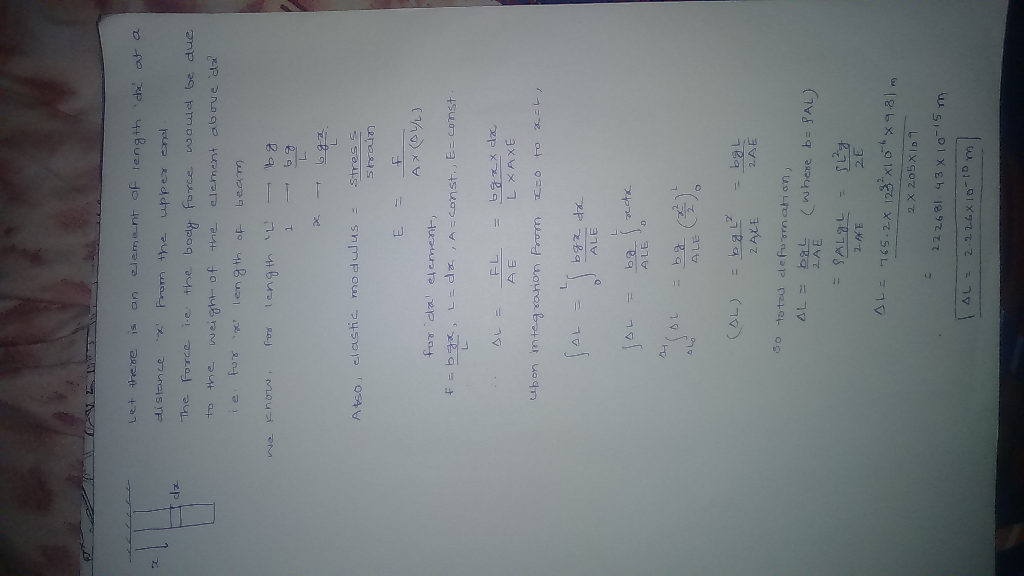#### Earn Coins

Coins can be redeemed for fabulous gifts.

Similar Homework Help Questions
• ### Determine the nodal displacements and reaction forces using the finite element direct method for the 1-D bar elements connected as shown below.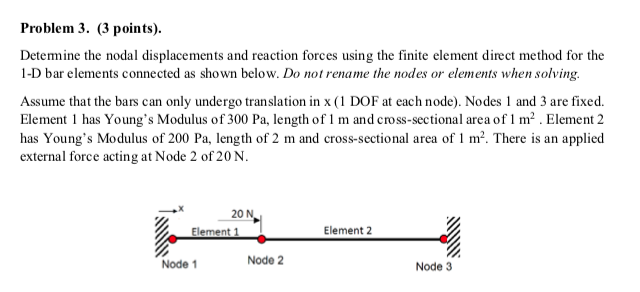Determine the nodal displacements and reaction forces using the finite element direct method for the 1-D bar elements connected as shown below. Do not rename the nodes or elements when solving. Assume that the bars can only undergo translation in x (1 DOF at each node). Nodes 1 and 3 are fixed Element 1 has Young's Modulus of 300 Pa, length of 1 m and cross-sectional area of 1 m2. Element 2 has Young's Modulus of 200 Pa, length of 2...

• ### Problem 3. (3 points). Determine the nodal displacements and reaction forces using the finite element direct...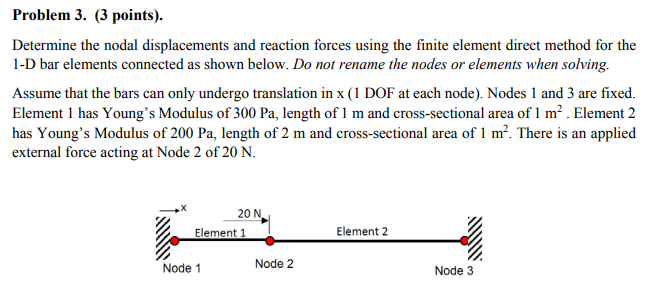Problem 3. (3 points). Determine the nodal displacements and reaction forces using the finite element direct method for the 1-D bar elements connected as shown below. Do not rename the nodes or elements when solving. Assume that the bars can only undergo translation in x (1 DOF at each node). Nodes 1 and 3 are fixed. Element 1 has Young's Modulus of 300 Pa, length of 1 m and cross-sectional area of m. Element 2 has Young's Modulus of 200...

• ### Solve the two problems below using the finite element method with Euler-Bernoulli beam element. 2...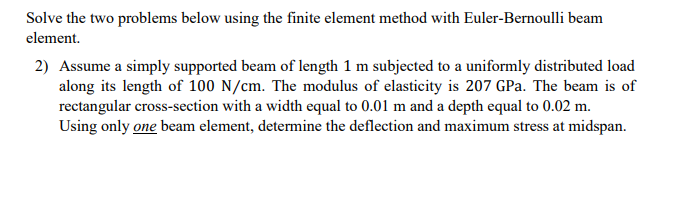Solve the two problems below using the finite element method with Euler-Bernoulli beam element. 2) Assume a simply supported beam of length 1 m subjected to a uniformly distributed load along its length of 100 N/cm. The modulus of elasticity is 207 GPa. The beam is of rectangular cross-section with a width equal to 0.01 m and a depth equal to 0.02 m. Using only one beam element, determine the deflection and maximum stress at midspan. Solve the two problems...

• ### CAN I HAVE A DETAILED EXPLANATION FOR ALL OF THEM ( ESPECIALLY A AND D )...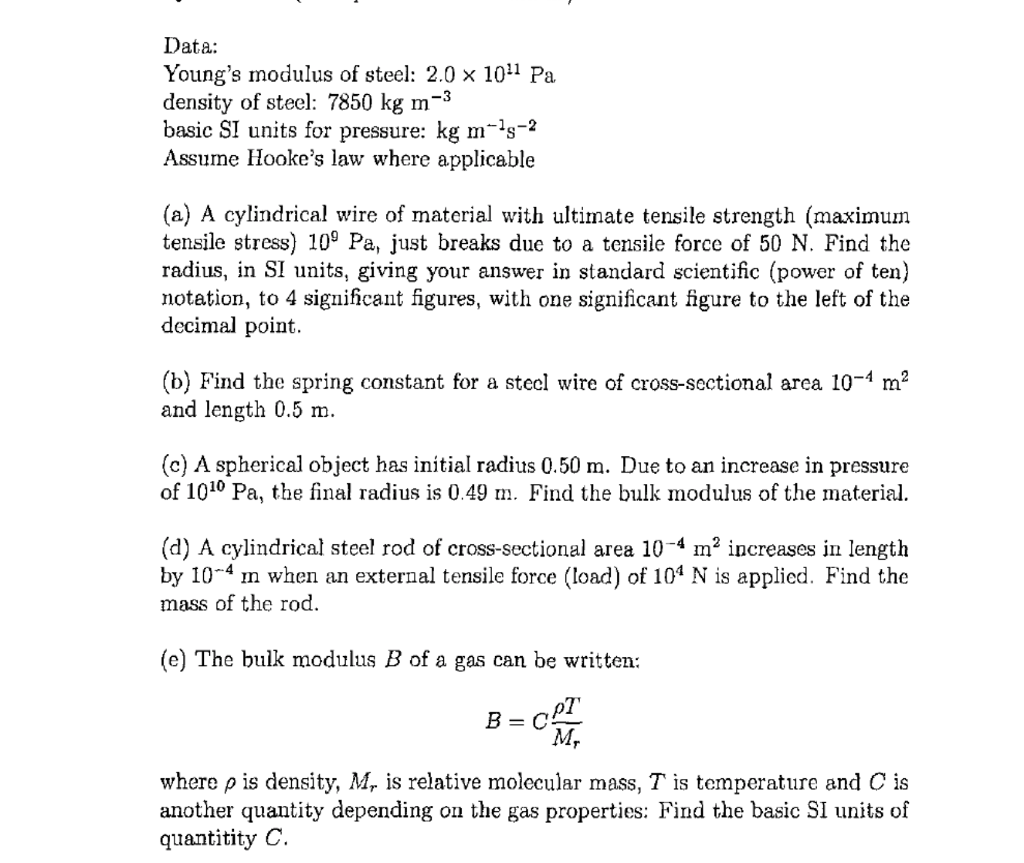CAN I HAVE A DETAILED EXPLANATION FOR ALL OF THEM ( ESPECIALLY A AND D ) PLEASE ( CLEAR HANDWRITING) Data: Young's modulus of steel: 2.0 × 1011 Pa density of steel: 7850 kg m-3 basic SI units for pressure: kg m-1s-2 Assume Hooke's law where applicable (a) A cylindrical wire of material with ultimate tensile strength (maximum tensile stress) 109 Pa, just breaks due to a tensile force of 50 N. Find the radius, in SI units, giving your...

• ### Section 1: Finite Element Derivation and Validation In this section of the report you will develop your own Finite Elem...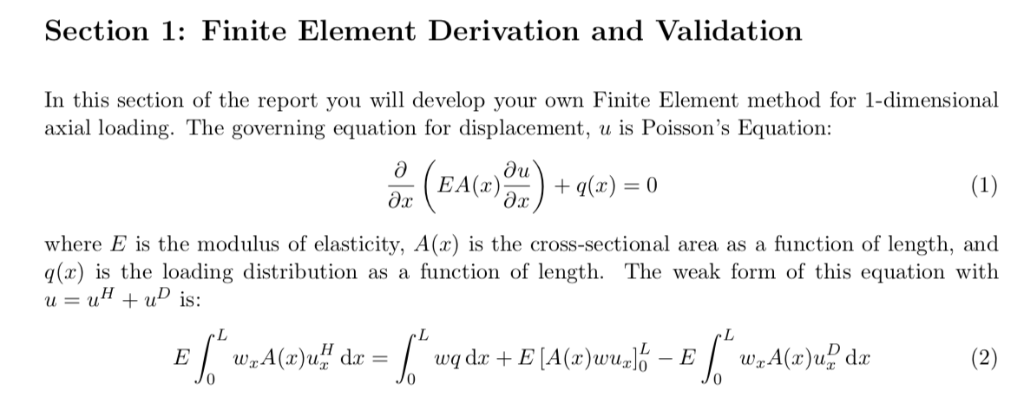Section 1: Finite Element Derivation and Validation In this section of the report you will develop your own Finite Element method for 1-dimensional axial loading. The governing equation for displacement, u is Poisson's Equation: อั1 where E is the modulus of elasticity, A(a) is the cross-sectional area as a function of length, and q(x) is the loading distribution as a function of length. The weak form of this equation with 0 1. Starting from the weak form of the governing...

• ### Section 1: Finite Element Derivation and Validation In this section of the report you will develop your own Finite Elem...Section 1: Finite Element Derivation and Validation In this section of the report you will develop your own Finite Element method for 1-dimensional axial loading. The governing equation for displacement, u is Poisson's Equation: อั1 where E is the modulus of elasticity, A(a) is the cross-sectional area as a function of length, and q(x) is the loading distribution as a function of length. The weak form of this equation with 0 1. Starting from the weak form of the governing...

• ### For the next two problems use the following information: A simply supported Douglas fir wood beam...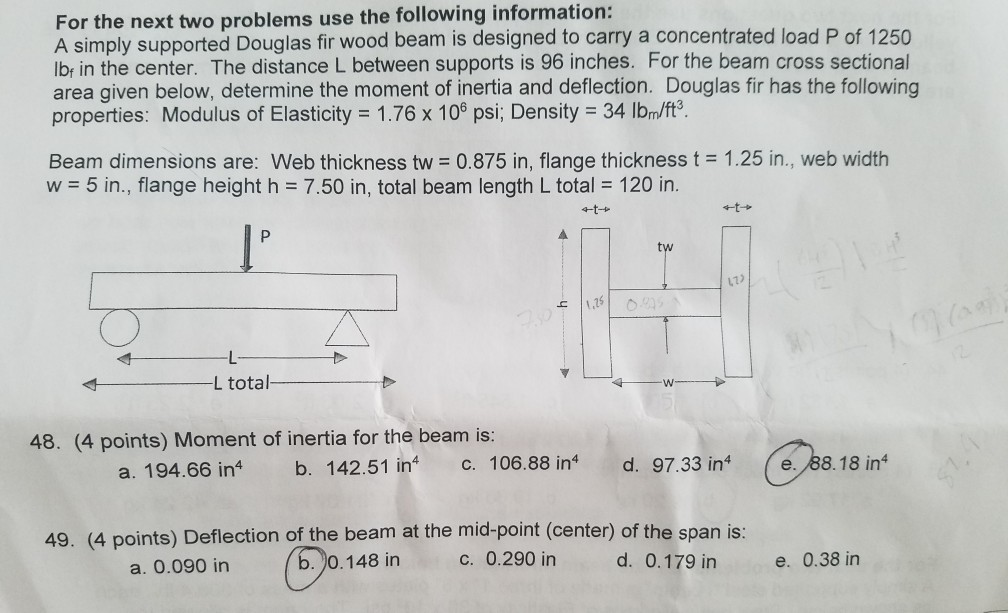For the next two problems use the following information: A simply supported Douglas fir wood beam is designed to carry a concentrated load P of 1250 lbr in the center. The distance L between supports is 96 inches. For the beam cross sectional area given below, determine the moment of inertia and deflection. Douglas fir has the following properties: Modulus of Elasticity 1.76 x 100 psi, Density 34 lbm/ff3 Beam dimensions are: Web thickness tw 0.875 in, flange thickness t...

• ### Q2 (a) (0) Explain what is meant by interpolation in the Finite Element Method and why...Q2 (a) (0) Explain what is meant by interpolation in the Finite Element Method and why it is used (3 marks) What is a shape function? (3 marks) PLEASE TURN OVER 16363,16367 Page 2 of 3 0.2 (a) (Continued) (iii) For an isoparametric element, explain the relationship between shape functions, the geometry of the element and the shape the loaded element will deform to. (3 marks) (iv) Describe the relationship between structural equilibrium and the minimum potential energy state. (3...

• ### A rigid beam BCD is supported on a roller support at C (4m from B) and has two bars AB and DE attached at each end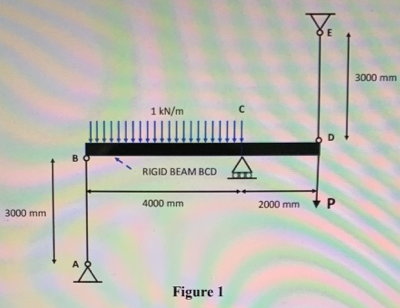A rigid beam BCD is supported on a roller support at C (4m from B) and has two bars AB and DE attached at each end. The bars can carry either tension or compressive forces. The rigid beam carries a UDL of I kN/m across BC and a point load of P at D as shown in the figure above. The length of the two bars is 3000 mm. The elastic modulus of both bars is 200 GPa and the...

• ### need to solve the mathematical model to prove that we can get the equations i   Q1...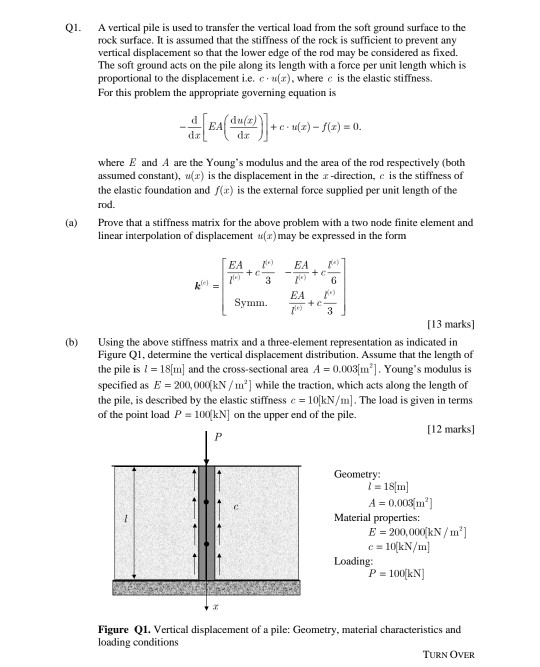need to solve the mathematical model to prove that we can get the equations i   Q1 a methematically QI. A vertical pile is used to transfer the vertical load from the soft ground surface to the rock surface. It is assumed that the stiffness of the rock is sufficient to prevent any vertical displacement so that the lower edge of the rod may be considered as fixed. The soft ground acts on the pile along its length with a force...